# 使用 ggplot2 和 RColorBrewer 扩展调色板

library(ggplot2)

ggplot(mtcars, aes(factor(cyl), fill=factor(cyl))) +
geom_bar()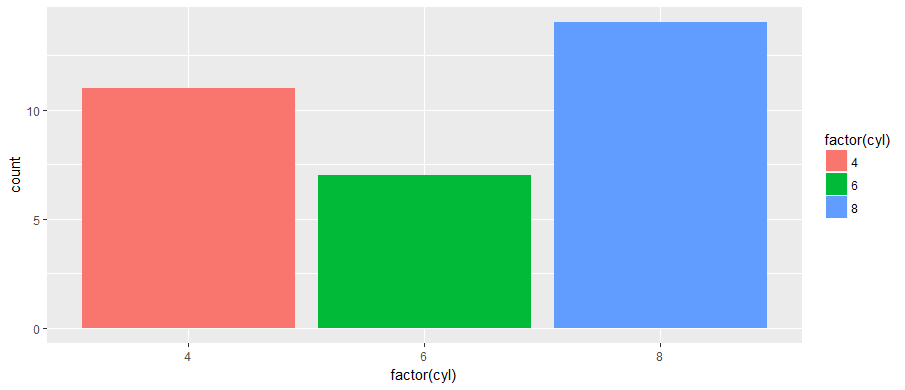ggplot函数的优雅在于对可视化公式简单而紧凑的表达，同时隐藏了默认情况下许多假设选项。隐藏并不意味着缺乏，因为大多数选择只是一步之遥。例如，对于颜色选择，使用scale函数族中的其中一种选择scale_fill_brewer():

ggplot(mtcars, aes(factor(cyl), fill=factor(cyl))) +
geom_bar() +
scale_fill_brewer()ggplot(mtcars, aes(factor(cyl), fill=factor(cyl))) +
geom_bar() +
scale_fill_brewer(palette="Set1")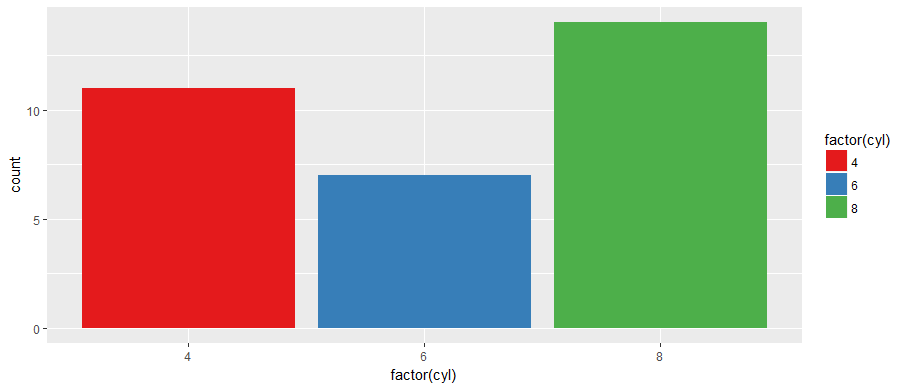Palettes在包RColorBrewer中 - 运行 display.brewer.all() 可以看到所有的选择：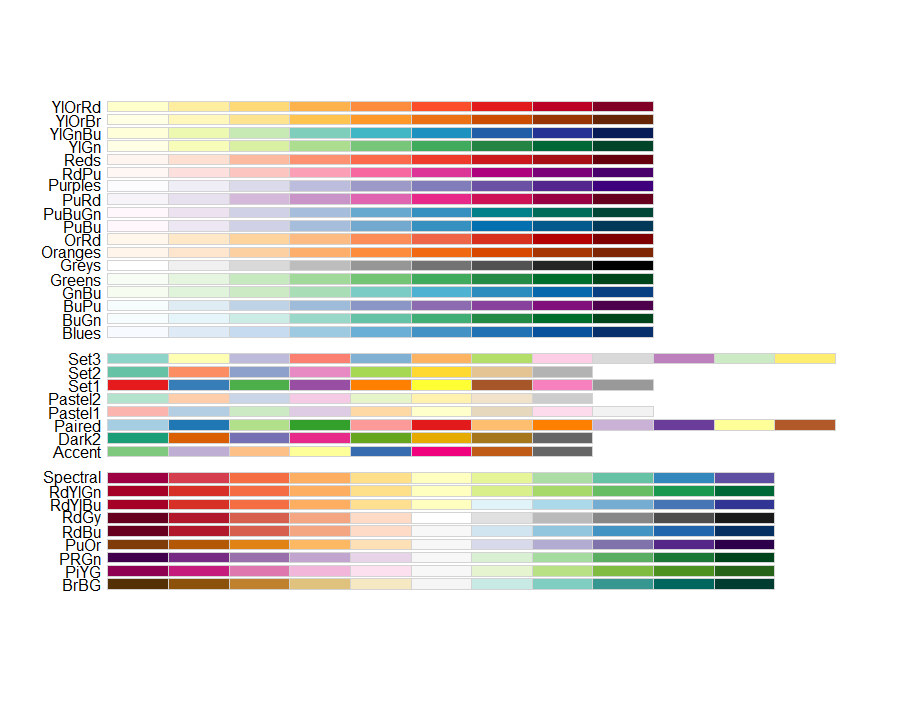brewer.pal.info 或者 ?RColorBrewer看到更多的细节）。

ggplot(mtcars) +
geom_bar(aes(factor(hp), fill=factor(hp))) +
scale_fill_brewer(palette="Set2")getPalette = colorRampPalette(brewer.pal(9, "Set1"))

ggplot(mtcars) +
geom_bar(aes(factor(hp)), fill=getPalette(colourCount)) +
theme(legend.position="right")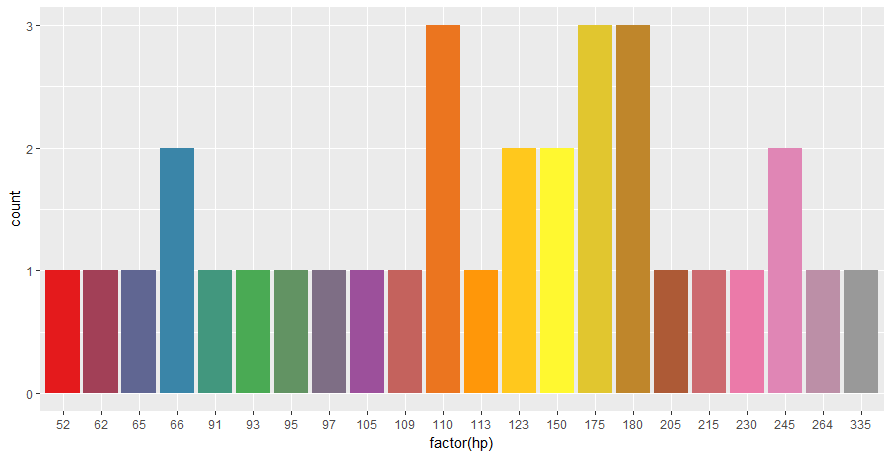ggplot(mtcars) +
geom_bar(aes(factor(hp), fill=factor(hp))) +
scale_fill_manual(values = getPalette(colourCount))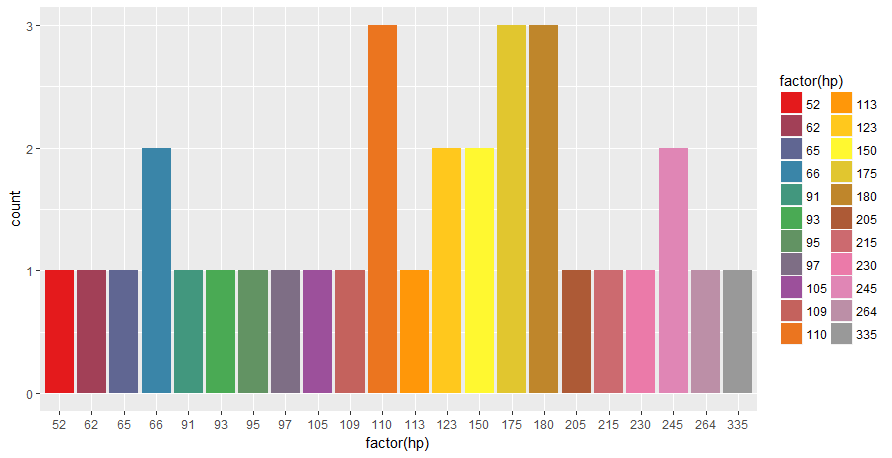ggplot(mtcars) +
geom_bar(aes(factor(hp), fill=factor(hp))) +
scale_fill_manual(values = getPalette(colourCount)) +
theme(legend.position="bottom") +
guides(fill=guide_legend(nrow=2))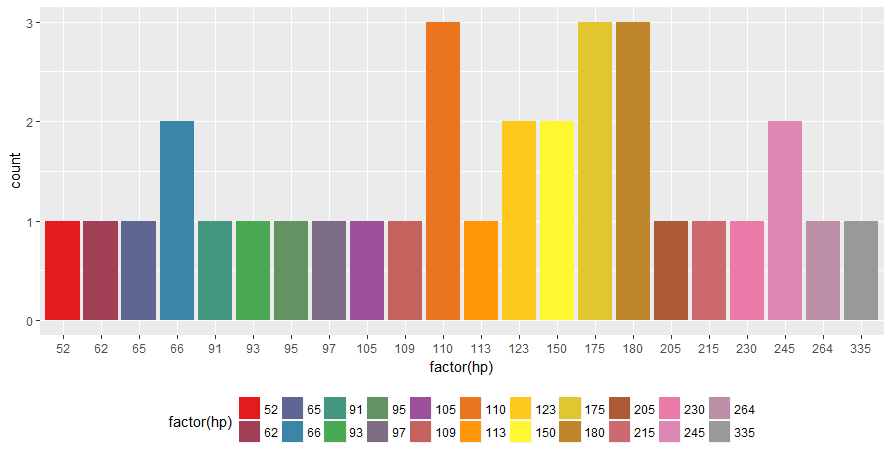ggplot(mtcars) +
geom_bar(aes(factor(hp), fill=factor(hp))) +
scale_fill_manual(values = colorRampPalette(brewer.pal(12, "Accent"))(colourCount)) +
theme(legend.position="bottom") +
guides(fill=guide_legend(nrow=2))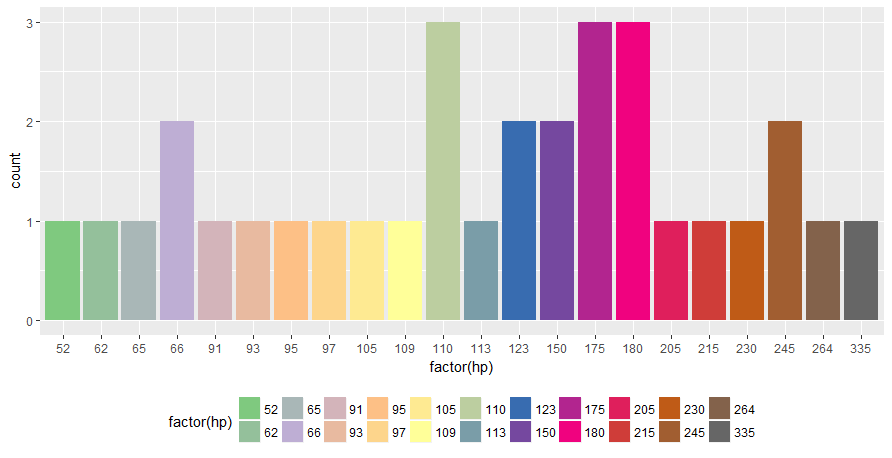posted @ 2018-09-06 19:09  Hiidiot  阅读(...)  评论(...编辑  收藏